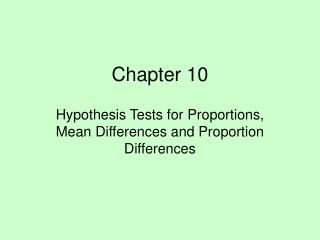# Chapter 10 - PowerPoint PPT PresentationDownload PresentationChapter 10

Chapter 10Download Presentation## Chapter 10

- - - - - - - - - - - - - - - - - - - - - - - - - - - E N D - - - - - - - - - - - - - - - - - - - - - - - - - - -
##### Presentation Transcript

1. Chapter 10 Hypothesis Tests for Proportions, Mean Differences and Proportion Differences

2. Figure 10.1 The Sampling Distribution of the Sample Proportion  = p

3.  = = .024 p = .06 Figure 10.2 The “Null” Sampling Distribution

4. 0 zc = 1.65 z REJECT H0 p= .06 Figure 10.3 Setting a Boundary on the Null Sampling Distribution a= .05

5. Test Statistic for a (10.1) Sample Proportion zstat =

6. REJECT H0 p = .06 z 0 zc = 1.65 Figure 10.4 Showing the Sample Result on the Null Sampling Distribution = .11 zstat = 2.08

7. REJECT H0 p = .06c = .099 z 0 zc = 1.65 Figure 10.5 Identifying the Critical

8. p-value=.0188 .4812 p = .06 = .11 Figure 10.6 Computing the p-value z 0 z = 2.08

9. s= m1 - m2 Figure 10.7 The Sampling Distribution of the Sample Mean Difference

10. m1 - m2 = 0 Figure 10.8 The “Null” Sampling Distribution

11. REJECT H0 REJECT H0 m1 - m2 = 0 z zcu= +1.96 zcl= -1.96 Figure 10.9 Setting Boundaries on the Null Sampling Distribution a/2 = .025 a/2 = .025 0

12. Test Statistic (10.2) (s values are known) zstat=

13. REJECT H0 REJECT H0 m1 - m2 = 0 z zcu= +1.96 zcl= -1.96 Figure 10.10 Showing zstaton the Null Sampling Distribution zstat= 2.51 0

14. Estimated Standard Error of the (10.3)Sampling Distribution of Mean Differences (large samples) =

15. Test Statistic for Large Samples, (10.4)s values unknown zstat =

16. Pooling Sample (10.6) Standard Deviations spooled =

17. Estimated Standard Error of the (10.7) Sampling Distribution of the Sample Mean Difference (small samples) =

18. Calculating tstat 1. Pool the sample standard deviations: 2. Estimate the standard error (standard deviation) of the sampling distribution: 3. Calculate the test statistic: tstat = Spooled= =

19. s = p1 -p2 Figure 10.11 The Sampling Distribution of the Sample Proportion Difference

20. p1 -p2 = 0 Figure 10.12 The “Null” Sampling Distribution

21. REJECT H0 a = .01 p1 -p2 = 0 zc = 2.33 z 0 Figure 10.13 Setting the Boundary on the Null Sampling Distribution

22. Pooling the Sample Proportions (10.9)

23. Estimated Standard Error (10.10) of the Null Sampling Distribution

24. REJECT H0 zstat = .877 p1-p2 = 0 z 0 zc = 2.33 Figure 10.14 Showingzstaton the Null Sampling Distribution

25. Test Statistic for Matched (10.11) Samples Case tstat=

26. Standard Deviation of the (10.12) Sample Mean Differences sd =